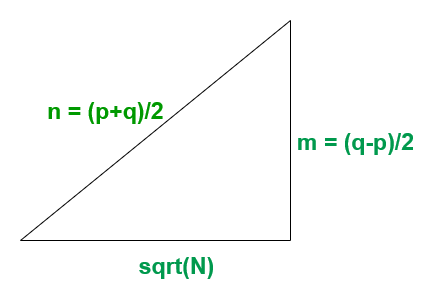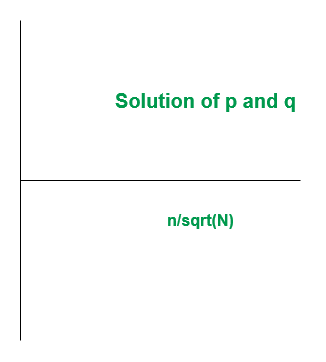# Check if a number is Prime, Semi-Prime or Composite for very large numbers

• Difficulty Level : Hard
• Last Updated : 21 Dec, 2021

Given a very large number N (> 150), the task is to check whether this number is Prime, Semi-Prime or Composite.
Example:

Input: N = 90000000
Output: Not Prime
Explanation:
we have (N-1)%6 = 89999999%6 = 1 and
(N+1)%6 = 90000001%6 = 5
Since n-1 and n+1 is not divisible by 6
Therefore N = 90000000 is Not Prime
Input: N = 7894561
Output: Semi-Prime
Explanation:
Here N = 7894561 = 71*111191
Since 71 & 111191 are prime, therefore 7894561 is Semi Prime

Approach:

• It can be observed that if n is a Prime Number then n+1 or n-1 will be divisible by 6
• If a number n exists such that neither n+1 nor n-1 is divisible by 6 then n is not a prime number
• If a number n exists such that either n+1 or n-1 is divisible by 6 then n is either a prime or a semiprime number
• To differentiate between prime and semi-prime, the following method is used:
• If N is semi prime then,
```N = p*q  ....................(1)
where p & q are primes.```
```p + q must be even
i.e, p + q = 2*n for any positive integer n```
• Therefore solving for p & q will give
```p = n - sqrt(n2 - N)
q = n + sqrt(n2 - N)```
• Let n2 – N be perfect square, Then
```n2 - N = m2, .................(2)
for any positive integer m ```
• Solving Equations (1) & (2) we get
```m = (q-p)/2
n = (p+q)/2```
• Now if equation (1) & (2) meets at some point, then there exists a pair (p, q) such that the number N is semiprime otherwise N is prime.
• Equation(2) forms Pythagorean Triplet• The solution expected varies on the graphPseudo code:

• Input a number N and if N – 1 and N + 1 is not divisible by 6 then the number N is Not Prime. else it is prime or semi-prime
• If n-1 or n+1 is divisible by 6 then iterate in the range(sqrt(N) + 1, N) and find a pair (p, q) such that p*q = N by below formula:
```p = i - sqrt(i*i - N)
q = n/p
where i = index in range(sqrt(N) + 1, N)```
• If p*q = N then the number N is semi prime, else it is prime

Below is the implementation of the above approach:

## Java

 `import` `static` `java.lang.Math.sqrt;` `public` `class` `Primmefunc {` `    ``public` `static` `void` `prime(``long` `n)``    ``{``        ``int` `flag = ``0``;` `        ``// checking divisibility by 6``        ``if` `((n + ``1``) % ``6` `!= ``0` `&& (n - ``1``) % ``6` `!= ``0``) {``            ``System.out.println(``"Not Prime"``);``        ``}``        ``else` `{` `            ``// breakout if number is perfect square``            ``double` `s = sqrt(n);``            ``if` `((s * s) == n) {``                ``System.out.println(``"Semi-Prime"``);``            ``}``            ``else` `{``                ``long` `f = (``long``)s;``                ``long` `l = (``long``)((f * f));` `                ``// Iterating over to get the``                ``// closest average value``                ``for` `(``long` `i = f + ``1``; i < l; i++) {` `                    ``// 1st Factor``                    ``long` `p = i - (``long``)(sqrt((i * i) - (n)));` `                    ``// 2nd Factor``                    ``long` `q = n / p;` `                    ``// To avoid Convergence``                    ``if` `(p < ``2` `|| q < ``2``) {``                        ``break``;``                    ``}` `                    ``// checking semi-prime condition``                    ``if` `((p * q) == n) {``                        ``flag = ``1``;``                        ``break``;``                    ``}` `                    ``// If convergence found``                    ``// then number is semi-prime``                    ``else` `{` `                        ``// convergence not found``                        ``// then number is prime``                        ``flag = ``2``;``                    ``}``                ``}` `                ``if` `(flag == ``1``) {``                    ``System.out.println(``"Semi-Prime"``);``                ``}``                ``else` `if` `(flag == ``2``) {` `                    ``System.out.println(``"Prime"``);``                ``}``            ``}``        ``}``    ``}` `    ``public` `static` `void` `main(String[] args)``    ``{``        ``// Driver code``        ``// Entered number should be greater``        ``// than 300 to avoid Convergence of``        ``// second factor to 1``        ``prime(``8179``);``        ``prime(``7894561``);``        ``prime(``90000000``);``        ``prime(``841``);``        ``prime(``22553``);``        ``prime(``1187``);``    ``}``//written by Rushil Jhaveri``}`

## CPP

 `#include``using` `namespace` `std ;` `void` `prime(``long` `n)``{``    ``int` `flag = 0;` `    ``// checking divisibility by 6``    ``if` `((n + 1) % 6 != 0 && (n - 1) % 6 != 0)``    ``{``        ``cout << (``"Not Prime"``) << endl;``    ``}``    ``else``    ``{` `        ``// breakout if number is perfect square``        ``double` `s = ``sqrt``(n);``        ``if` `((s * s) == n)``        ``{``            ``cout<<(``"Semi-Prime"``)<

## Python3

 `def` `prime(n):``    ``flag ``=` `0``;` `    ``# checking divisibility by 6``    ``if` `((n ``+` `1``) ``%` `6` `!``=` `0` `and` `(n ``-` `1``) ``%` `6` `!``=` `0``):``        ``print``(``"Not Prime"``);``    ``else``:` `        ``# breakout if number is perfect square``        ``s ``=` `pow``(n, ``1``/``2``);``        ``if` `((s ``*` `s) ``=``=` `n):``            ``print``(``"Semi-Prime"``);``        ``else``:``            ``f ``=` `int``(s);``            ``l ``=` `int``(f ``*` `f);` `            ``# Iterating over to get the``            ``# closest average value``            ``for` `i ``in` `range``(f ``+` `1``, l):` `                ``# 1st Factor``                ``p ``=` `i ``-` `(``pow``(((i ``*` `i) ``-` `(n)), ``1``/``2``));` `                ``# 2nd Factor``                ``q ``=` `n ``/``/` `p;` `                ``# To avoid Convergence``                ``if` `(p < ``2` `or` `q < ``2``):``                    ``break``;``                ` `                ``# checking semi-prime condition``                ``if` `((p ``*` `q) ``=``=` `n):``                    ``flag ``=` `1``;``                    ``break``;``                ` `                ``# If convergence found``                ``# then number is semi-prime``                ``else``:` `                    ``# convergence not found``                    ``# then number is prime``                    ``flag ``=` `2``;``                ` `            ``if` `(flag ``=``=` `1``):``                ``print``(``"Semi-Prime"``);``            ``elif``(flag ``=``=` `2``):` `                ``print``(``"Prime"``);``            ` `# Driver code``if` `__name__ ``=``=` `'__main__'``:` `    ``# Entered number should be greater``    ``# than 300 to avoid Convergence of``    ``# second factor to 1``    ``prime(``8179``);``    ``prime(``7894561``);``    ``prime(``90000000``);``    ``prime(``841``);``    ``prime(``22553``);``    ``prime(``1187``);` `# This code is contributed by 29AjayKumar`

## C#

 `using` `System;``public` `class` `Primmefunc``{` `    ``public` `static` `void` `prime(``long` `n)``    ``{``        ``int` `flag = 0;` `        ``// checking divisibility by 6``        ``if` `((n + 1) % 6 != 0 && (n - 1) % 6 != 0)``        ``{``            ``Console.WriteLine(``"Not Prime"``);``        ``}``        ``else``        ``{` `            ``// breakout if number is perfect square``            ``double` `s = Math.Sqrt(n);``            ``if` `((s * s) == n)``            ``{``                ``Console.WriteLine(``"Semi-Prime"``);``            ``}``            ``else``            ``{``                ``long` `f = (``long``)s;``                ``long` `l = (``long``)((f * f));` `                ``// Iterating over to get the``                ``// closest average value``                ``for` `(``long` `i = f + 1; i < l; i++)``                ``{` `                    ``// 1st Factor``                    ``long` `p = i - (``long``)(Math.Sqrt((i * i) - (n)));` `                    ``// 2nd Factor``                    ``long` `q = n / p;` `                    ``// To avoid Convergence``                    ``if` `(p < 2 || q < 2)``                    ``{``                        ``break``;``                    ``}` `                    ``// checking semi-prime condition``                    ``if` `((p * q) == n)``                    ``{``                        ``flag = 1;``                        ``break``;``                    ``}` `                    ``// If convergence found``                    ``// then number is semi-prime``                    ``else``                    ``{` `                        ``// convergence not found``                        ``// then number is prime``                        ``flag = 2;``                    ``}``                ``}` `                ``if` `(flag == 1)``                ``{``                    ``Console.WriteLine(``"Semi-Prime"``);``                ``}``                ``else` `if` `(flag == 2)``                ``{``                    ``Console.WriteLine(``"Prime"``);``                ``}``            ``}``        ``}``    ``}` `    ``// Driver code``    ``public` `static` `void` `Main(String[] args)``    ``{``        ``// Entered number should be greater``        ``// than 300 to avoid Convergence of``        ``// second factor to 1``        ``prime(8179);``        ``prime(7894561);``        ``prime(90000000);``        ``prime(841);``        ``prime(22553);``        ``prime(1187);``    ``}``}` `// This code is contributed by 29AjayKumar`

## Javascript

 ``
Output:
```Prime
Semi-Prime
Not Prime
Semi-Prime
Semi-Prime
Prime```

Time Complexity: O(N)

My Personal Notes arrow_drop_up